# Compound Interest

## Basic Formulas of Compound Interest

If A = Amount
P = Principle
C.I. = Compound Interest
T = Time in years
R = Interest Rate Per Year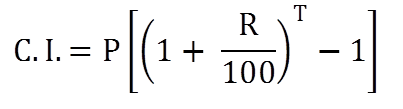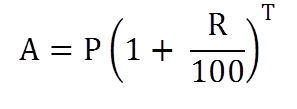## Shortcut Formulas for Compound Interest

Rule 1: If rate of interest is R1% for first year, R2% for second year and R3% for third year, thenRule 2:
If principle = P, Rate = R% and Time = T years then

1. If the interest is compounded annually:2. If the interest is compounded half yearly (two times in year):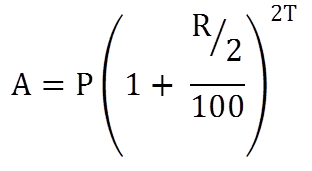3. If the interest is compounded quarterly (four times in year):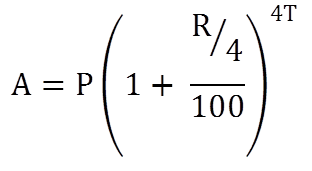Rule 3: If difference between Simple Interest and Compound Interest is given.

• If the difference between Simple Interest and Compound Interest on a certain sum of money for 2 years at R% rate is given then• If the difference between Simple Interest and Compound Interest on a certain sum of money for 3 years at R% is given then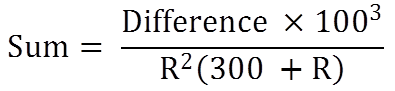Rule 4: If sum A becomes B in T1 years at compound interest, then after T2 years